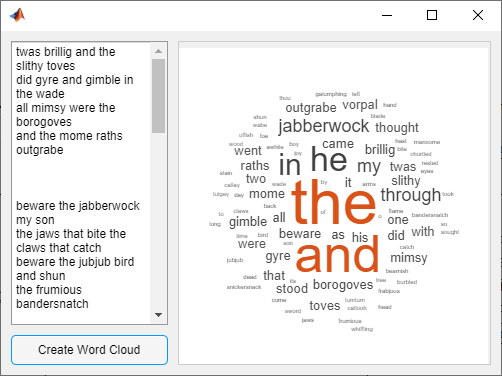## 在回调之间共享数据

### 存储 App 数据

App 中的 UI 组件在其属性中包含了有用的信息。例如，可以通过查询滑块的 `Value` 属性来查找滑块的当前位置。创建 UI 组件时，请将该组件存储为变量，以便您可以在整个 App 代码中设置和访问其属性。

```fig = uifigure; d = uidatepicker(fig); date = datetime("today"); fig.UserData = struct("Datepicker",d,"Today",date);```

### 从回调函数访问 App 数据

• 访问 UserData 中的数据 - 使用此方法从回调函数中更新 App 数据。它要求您将 App 数据存储在 `UserData` 属性中，如前一节中所述。

• 将输入数据传递给回调 - 在简单的 App 中使用此方法来限制回调可以访问的数据，并且可以更轻松地重用回调代码。

• 创建嵌套回调函数 - 在简单的 App 中使用此方法，让您的回调函数可以访问所有 App 数据，并在单个文件中组织您的 App 代码。### 访问 `UserData` 中的数据

`fig = ancestor(btn,"figure","toplevel");`

#### 示例：使用 `UserData` 的文字云

`fig.UserData = struct("TextArea",txt,"Panel",pnl);`

`createWordCloud` 函数中，访问图窗中的 `UserData` 属性。由于 MATLAB 会自动将执行回调的组件作为 `src` 传递给回调函数，您可以使用 `ancestor` 函数从回调函数访问该图窗。

`fig = ancestor(src,"figure","toplevel");`

```data = fig.UserData; txt = data.TextArea; pnl = data.Panel; val = txt.Value;```

```function shareUserData % Create figure and grid layout fig = uifigure; gl = uigridlayout(fig,[2,2]); gl.RowHeight = {'1x',30}; gl.ColumnWidth = {'1x','2x'}; % Create and lay out text area txt = uitextarea(gl); txt.Layout.Row = 1; txt.Layout.Column = 1; % Create and lay out button btn = uibutton(gl); btn.Layout.Row = 2; btn.Layout.Column = 1; btn.Text = "Create Word Cloud"; % Create and lay out panel pnl = uipanel(gl); pnl.Layout.Row = [1 2]; pnl.Layout.Column = 2; % Store data in figure fig.UserData = struct("TextArea",txt,"Panel",pnl); % Assign button callback function btn.ButtonPushedFcn = @createWordCloud; end % Process and plot text function createWordCloud(src,event) fig = ancestor(src,"figure","toplevel"); data = fig.UserData; txt = data.TextArea; pnl = data.Panel; val = txt.Value; words = {}; for k = 1:length(val) text = strsplit(val{k}); words = [words text]; end c = categorical(words); wordcloud(pnl,c); end```

### 将输入数据传递给回调

#### 示例：使用回调输入参数的文字云应用

```function createWordCloud(src,event,txt,pnl) % Code to plot the word cloud end```

`createWordCloud` 回调函数赋值，并在文本区域和面板中传递，方法是将 `ButtonPushedFcn` 指定为包含 `createWordCloud` 句柄的元胞数组，后跟其他输入参数。

`btn.ButtonPushedFcn = {@createWordCloud,txt,pnl};`

```function shareAsInput % Create figure and grid layout fig = uifigure; gl = uigridlayout(fig,[2,2]); gl.RowHeight = {'1x',30}; gl.ColumnWidth = {'1x','2x'}; % Create and lay out text area txt = uitextarea(gl); txt.Layout.Row = 1; txt.Layout.Column = 1; % Create and lay out button btn = uibutton(gl); btn.Layout.Row = 2; btn.Layout.Column = 1; btn.Text = "Create Word Cloud"; % Create and lay out panel pnl = uipanel(gl); pnl.Layout.Row = [1 2]; pnl.Layout.Column = 2; % Assign button callback function btn.ButtonPushedFcn = {@createWordCloud,txt,pnl}; end % Process and plot text function createWordCloud(src,event,txt,pnl) val = txt.Value; words = {}; for k = 1:length(val) text = strsplit(val{k}); words = [words text]; end c = categorical(words); wordcloud(pnl,c); end```

### 创建嵌套回调函数

#### 示例：使用嵌套回调的文字云应用

```function nestCallback % Create figure and grid layout fig = uifigure; gl = uigridlayout(fig,[2,2]); gl.RowHeight = {'1x',30}; gl.ColumnWidth = {'1x','2x'}; % Create and lay out text area t = uitextarea(gl); t.Layout.Row = 1; t.Layout.Column = 1; % Create and lay out button b = uibutton(gl); b.Layout.Row = 2; b.Layout.Column = 1; b.Text = "Create Word Cloud"; % Create and lay out panel p = uipanel(gl); p.Layout.Row = [1 2]; p.Layout.Column = 2; % Assign button callback function b.ButtonPushedFcn = @createWordCloud; % Process and plot text function createWordCloud(src,event) val = t.Value; words = {}; for k = 1:length(val) text = strsplit(val{k}); words = [words text]; end c = categorical(words); wordcloud(p,c); end end```